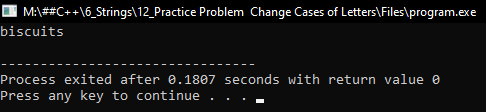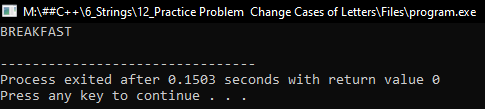# How to Change Cases of Letters of a String in C++

## How to Change Cases of Letters of a String in C++:

In this article, I am going to discuss How to Change Cases of Letters of a String in C++ Language with examples. Please read our previous article, where we discussed How to Find the Length of a String in C++ with examples.

##### How to Change Cases of Letters of a String in C++?

Here, we will write a program that will change the cases of letters of the given string. Let us see the program.

##### Program 1: Upper case to Lower case.
```#include <iostream>
#include <string.h>
using namespace std;
int main()
{
string str = "BISCUITS";
for (int i = 0; str[i] != '\0'; i++)
{
if (str[i] >= 65 && str[i] <= 90)
{
str[i] = str[i] + 32;
}
}
cout << str << endl;
return 0;
}
```
###### Output:##### Program 2: Lower case to Upper case.
```#include <iostream>
#include <string.h>
using namespace std;
int main()
{
string str = "breakfast";
for (int i = 0; str[i] != '\0'; i++)
{
if (str[i] >= 97 && str[i] <= 122)
{
str[i] = str[i] - 32;
}
}
cout << str << endl;
return 0;
}
```
###### Output: